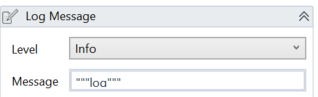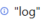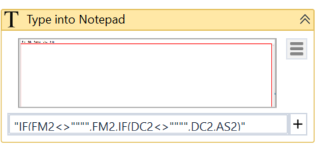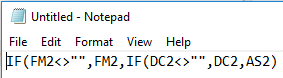# Write line: Excel formula for empty cells

Hi all,

I’m struggling with the syntaxes to add a formula in excel via the activity “write line”.
the formula looks like this: IF(FM2<>“”,FM2,IF(DC2<>“”,DC2,AS2)
How do I get tis right in UIPath? Because the “” is also UIPath language. Do i need to add single ’ somewhere?

Hey there.

First of all I don’t know if I misunderstood what you’re trying to do but the write line activity is used for debug. It writes a line in the output pane of your UiPath Studio.

Second of all you can escape double quotes in UiPath by using double quotation marks in your log or your write lineOutput :Example : I wrote in notepad your formula using a type text activityNote that I used the double quotation for each quote, meaning I typed

IF(FM2<>“”“”,FM2,IF(DC2<>“”“”,DC2,AS2)

IF(FM2<>“”,FM2,IF(DC2<>“”,DC2,AS2)

Result :As you can see it maintained the double quotes.

I used it for notepad but it should work the same when you type into Excel.

Hope this helped.

MANDOC Cosmin

First of all, sorry for my mistake - I am obviously using write cell and not write line.

I get an error while running it now: 'the range does not exist" - and I’m not sure if it is because my formula in the ‘value’ field of the write cell activity is wrong. Everything else seems to be fine…

It’s a little more complex then i first told, because I also have a variable nested in it (the variable is intRow which I convert to string).I don’t know what I am doing wrong, must be something with the double quotes or +.

This is the code I have entered:
“=IF(FM”+intRow.ToString+“<>”“”“,FM”+intRow.ToString+“,IF(DC”+intRow.ToString+“<>”“”“,DC”+intRow.ToString+“,AS”+intRow.ToString)

I started with an excel application scope and reading the range, storing the output as dt.
Any idea what could be the issue?

I don’t think the problem could be the value since that’s the value that you’re inserting into the cell, the problem is that UiPath can’t find the range of your cell.

First: Are you sure the problem comes from the write cell and not the read range? When your UiPath Robot crashes, are you sent to the Write Cell activity or the Read Range?

Second: Did you try checking the range of your Write Cell?

Could you possibly provide a screenshot of only the faulty activity?

The only time I got a “the range does not exist” error was when I accidentally put “A0” as the range value ^^

1 Like
1. Yes, the problem lies in the write cell as I am redirected to the write cell activity when the UIPath bot crashes.
2. the range of the cell I am writing to seems correct to me (see below). Column OE exists in the datatable.

1. the error message below:

Thanks a lot for taking your time!

@yannip

Before proceeding further, I noticed that your formula is missing a parenthesis at the end “)” (I typed it into Excel and it automatically corrected this:
`=IF(FM2<>"";FM2;IF(DC2<>"";DC2;AS2)`
to this:
`=IF(FM2<>"";FM2;IF(DC2<>"";DC2;AS2))`

First thought then would be that the formula is not accepted by the Excel, which in turn throws an exception and that Excel exception is then propagated as an error in UiPath.

Your formula here has same issue, you never close the first IF statement:

Could you give the fix a try first?

1 Like

Thanks a bunch! That did the trick!! I knew it had to be something very silly.

Also big thanks for getting me in the good direction!!

Hi everyone,
I am also facing a similar issue where I am trying to apply a formula in excel using write cell activity.
Below is the formula
=IF(B1=“000000”," “,IF(B1=” “,”", VALUE(C1))))

I am getting error end of expression missing
I have tried replacing double quotes with Chr(34) but it did not help.